# CW-complex

## Inductive definition

A CW-complex is a topological space$X$ constructed inductively as follows. We start with the$-1$-skeleton, which is empty. The$n$-skeleton, denoted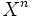$X^n$, is constructed from the$(n-1)$-skeleton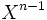$X^{n-1}$, by attaching a discretely parametrized family of attaching maps from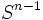$S^{n-1}$ to$X^{n-1}$, and taking the pushout with respect to these, for the inclusion of$S^{n-1}$ in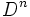$D^n$.

The space$X$ is given the union topology for the ascending unions of the skeleta. Each$n$-skeleton is closed in$X$, but not necessarily open in$X$.

A somewhat more general notion than a CW-complex is a cell complex, where we again attach cells, but it is now possible to attach the cells in any order rather than in the order of increasing dimension.

## Definition (assuming Hausdorffness)

A CW-complex is the following data, subject to the following conditions.

### Data

An ordered triple$(X,E,\Phi)$ where:

•$X$ is a Hausdorff space
•$E$ is a set of cells in$X$
•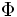$\Phi$ is a family of maps parametrized by the members of$E$

### Conditions

•$X$ is the disjoint union of all cells in$E$
• For each$k$-cell$e \in E$, the map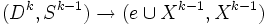$(D^k,S^{k-1}) \to (e \cup X^{k-1}, X^{k-1})$ is a relative homeomorphism
• The closure of any cell in$E$ is contained in a finite union of cells in$E$
•$X$ has the weak topology determined by the closures of the cells in$E$

### Terminology

•$X$ is termed a CW-space
•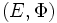$(E,\Phi)$ is called a CW-decomposition of$X$
•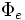$\Phi_e$ is termed the characteristic map of$e$